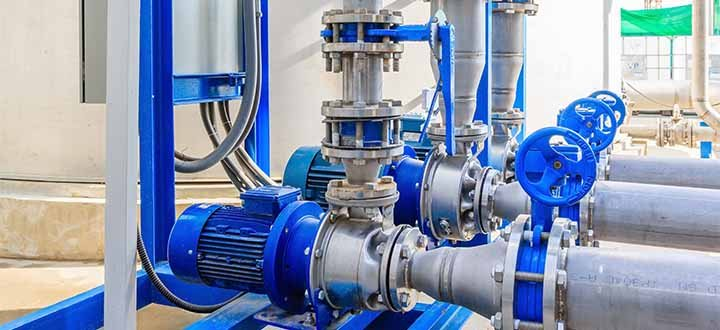PID control

PID control is used to adjust a controlled variable (e.g. pressure, temperature, frequency, etc.) to the setpoint. For pump control, these PID controllers are of great importance because they are an integrated component that controls the speed of the pump and thus enables automatic operation with all the resulting advantages.Functionality PID

The PID controller uses three elements, the P element (proportional component), the I element (integral component) and D element (differential component). The proportional component controls on the basis of the difference between the setpoint and the actual value.

The closer the actual value approaches the setpoint, the lower the frequency of the pump is controlled. Theoretically, the setpoint is never reached. The integral part solves the problem that the actual value approaches the setpoint, but does not reach it, because the correction signal is large enough even for small differences between the setpoint and actual value. The integral part therefore integrates the difference.

The differential component briefly amplifies the signal of the difference between the actual and setpoint values, so that the response of the controller is briefly accelerated. The D component is only recommended if the desired result is not achieved with the PI control.

Universal controller

The PID controller is also called a universal controller because of its wide range of applications and differentiated mode of operation. It can be adapted very well to different controlled systems and is thus a solution for diverse challenges.

If all links are used, the PID controller is characterized by a fast response to differences (due to the D component), a targeted start-up (due to the P component) and a precise control close to the setpoint (due to the I component). The control behavior is accordingly divided into three areas.